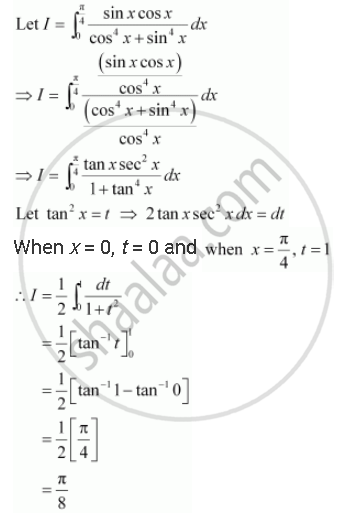Share

# Evaluate the Definite Integrals Int_0^(Pi/4) (Sinx Cos X)/(Cos^4 X + Sin^4 X)Dx - CBSE (Commerce) Class 12 - Mathematics

ConceptDefinite Integral as the Limit of a Sum

#### Question

Evaluate the definite integrals int_0^(pi/4) (sinx cos x)/(cos^4 x + sin^4 x)dx

#### SolutionIs there an error in this question or solution?

#### Video TutorialsVIEW ALL 

Solution Evaluate the Definite Integrals Int_0^(Pi/4) (Sinx Cos X)/(Cos^4 X + Sin^4 X)Dx Concept: Definite Integral as the Limit of a Sum.
S